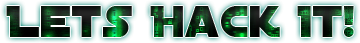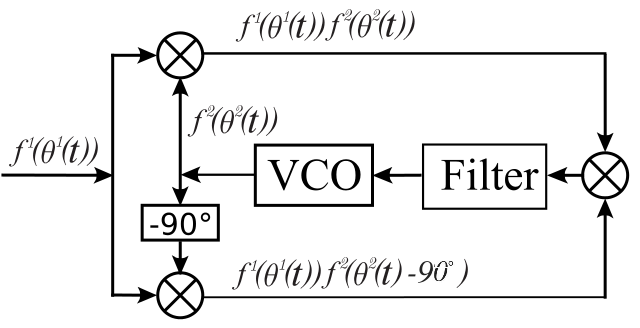# Demodulating BPSK Signal

How to make the reverse process (a.k.a. demodulate the signal)?

First we need to make sure that we have our carrier in the base band (a.k.a. center of our spectrum). You might think that just tunning at 1691MHz will make sure of that, but there are some factors that we need to account for:

1. The SDR Tuner Cristal is not 100% precise, and there will be some deviation from the actual signal.
2. The temperature change over the SDR will also lead to deviation on the signal.
3. Wind, atmosphere and other factors can doppler shift the signal.

How to do it then? We need to find where our signal really is, and translate to the base band (center of the spectrum). But we have a problem: The LRIT signal doesn’t actually contains the carrier wave, just the modulated signal (so its a signal that is a consequence of the modulated carrier wave).

If we had a Carrier Wave in the signal we could use a simple PLL (Phase Locked Loop) to track the carrier wave and translate the signal. But Carrier Waves also consume transmission power so the total transmission power (from the satellite) would be “wasted” in a signal just to track. For carrier-less signals like LRIT we need to use a more complex PLL system. There are several that can do that, but I will use the famous Costas Loop as suggested by Trango in #hearsat.Costas Loop is basically a double PLL system. The main idea is: If our supposed carrier is not centered, there will be a phase error that will accumulate over the time in the Quadrature Signal. So what we do? Use this error to translate our signal to the base band. The actual details of how the costas loop works will not be discussed here, but there are plenty of documents explaining how it works in the internet. For a BPSK we will use a 2nd Order Costas Loop. There is also the 3rd order costas loop for QPSK and 4th order costas loop for 8-PSK.

Ok, after that we need to recovery a second information: The Symbol Clock. The thing is: we modulated a binary signal over the carrier wave, but if we have like a string of 10 bits one, how we would know that the string has 10 bits, and not 5 or 7? We will need to recover the original clock using a M&M (Mueller and Müller) algorithm. But before that, how can we even recovery such thing?

Why we can recovery the original data clock?

We can recovery the original clock because we have two information:

1. The estimated symbol rate (in this case 293883 symbols / s)
2. The carrier phase transitions between 0 to 1 and 1 to 0

How can we recovery with just that information? Simple, we can do a oscillator (clock generator) in the estimated symbol rate, and synchronize with one carrier phase transitions. Then we can assume that we have a synchronized clock. Also because of the randomization of the data (that will be shown further in this article), the binary data will almost be random, that means that we will have basically the same odds to have bit 1 or 0. This will make our clock sync more consistent over time. Also M&M do an additional thing that is the clock frequency correction. Since the symbol rate is just an estimate, that means the value can change a little over time so M&M can correct that for us.

After that we should have our symbol in the I vector of our IQ Sample.